# Exercise 14.1 Symmetry -NCERT Solutions Class 7

## Chapter 14 Ex.14.1 Question 1

Copy the figures with punched holes and find the axes of symmetry for the following: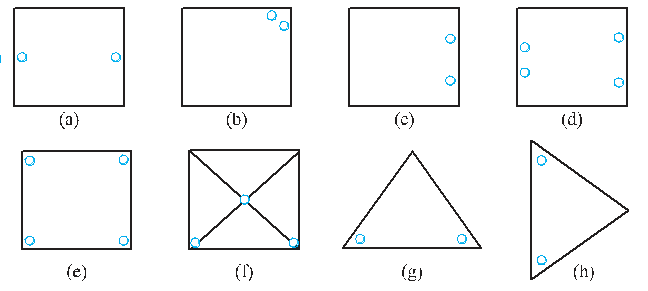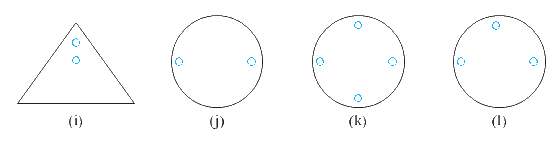### Solution

What is known?

The figure with punched holes.

What is unknown?

The axis of symmetry.

Reasoning:

This question is very simple. You have to draw different lines and check which line will divide it into exactly same parts, that line will be called the line of symmetry.

Steps: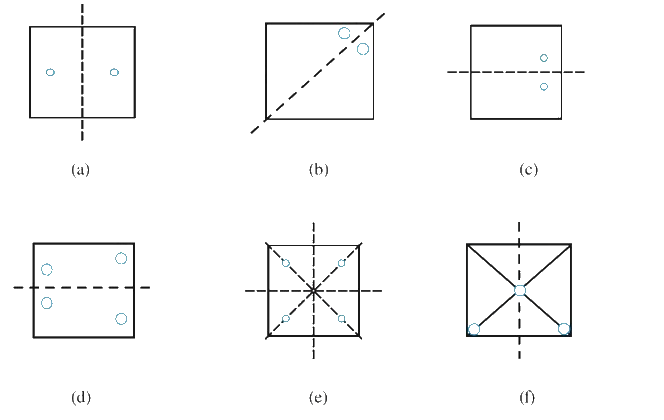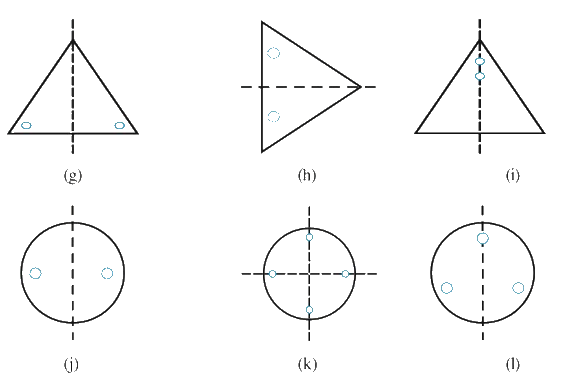(a) It has one line of symmetry.

(b) It has one line of symmetry.

(c) It has one line of symmetry.

(d) It has one line of symmetry.

(e) It has four lines of symmetry.

(f) It has one line of symmetry.

(g) It has one line of symmetry.

(h) It has one line of symmetry.

(i) It has one line of symmetry.

(j) It has one line of symmetry.

(k) It has two lines of symmetry.

(l) It has one line of symmetry.

## Chapter 14 Ex.14.1 Question 2

Given the line(s) of symmetry, find the other hole(s):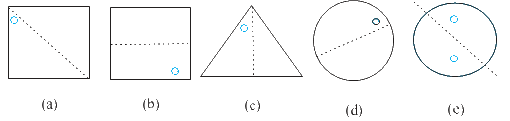### Solution

What is known?

The line of symmetry and the one hole

What is unknown?

The other hole.

Reasoning:

In this question a line of symmetry and a hole is given, you have to find the other hole. The other hole should be at that place such that if it is divided by any line of symmetry, it will divide it in equal parts.

Steps: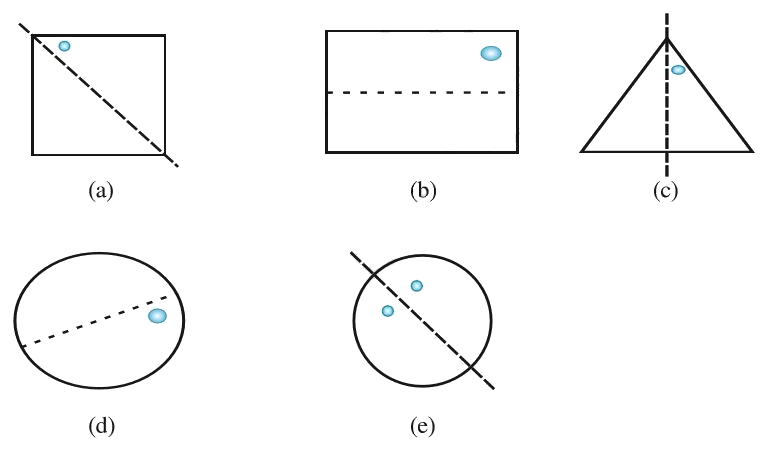## Chapter 14 Ex.14.1 Question 3

In the following figures, the mirror line (i.e., the line of symmetry) is given as a dotted line. Complete each figure performing reflection in the $$Q$$ dotted (mirror) line. (You might perhaps place a mirror along the dotted line and look into the mirror for the image). Are you able to recall the name of the figure you complete?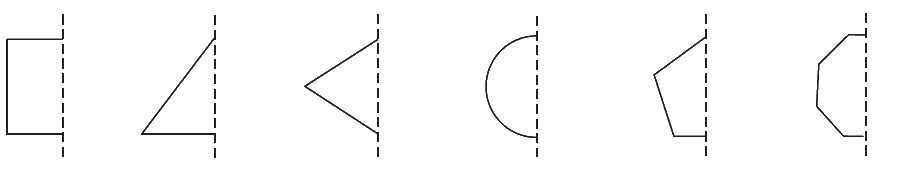### Solution

What is known?

The mirror line (i.e. the line of symmetry) is given as a dotted line.

What is unknown?

Complete each figure performing reflection in the dotted (mirror) line and also recall the name of the figure you complete.

Reasoning:

In this question you have to complete the figure, performing reflection in the dotted (mirror) line. To solve this question, you might perhaps place a mirror along the dotted line and look into the mirror for the image. By doing this you can get the hint which figure is formed and how to complete it.

Steps: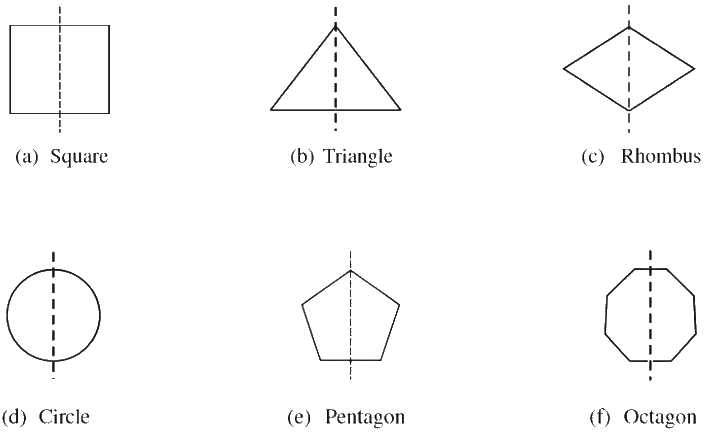(a) The shape formed is a square.

(b) The shape formed is a triangle.

(c) The shape formed is a rhombus.

(d) The shape formed is a circle.

(e) The shape formed is a pentagon.

(f) The shape formed is an octagon.

## Chapter 14 Ex.14.1 Question 4

The following figures have more than one line of symmetry. Such figures are said to have multiple lines of symmetry.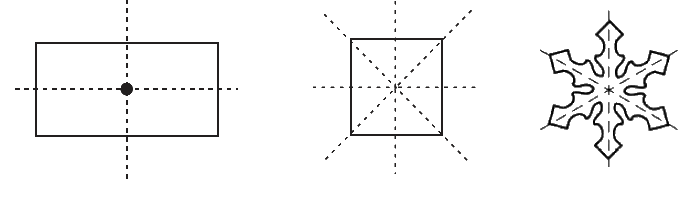Identify multiple lines of symmetry, if any, in each of the following figures: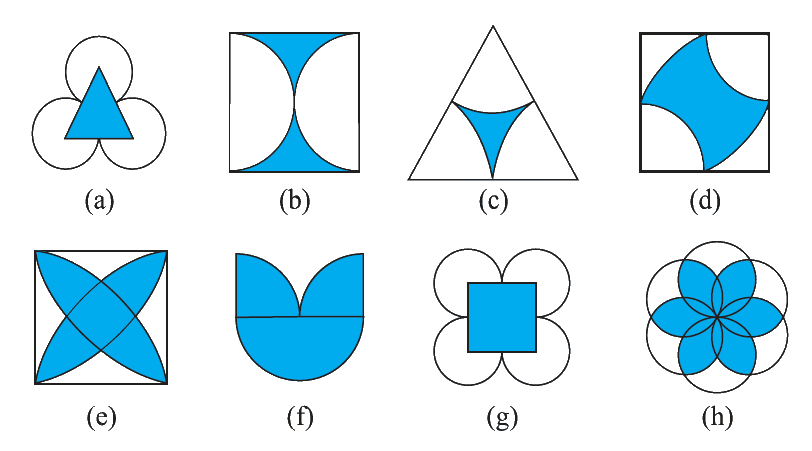### Solution

What is known?

Different figures.

What is unknown?

Multiple lines of symmetry.

Reasoning:

In this question you have to identify multiple of lines of symmetry. For this cut the figure by using different lines, the line which cuts the figure in two same parts are called lines of symmetry and a figure has more than one line of symmetry then we can say that it has multiple lines of symmetry.

Steps:(a) It has three lines of symmetry.

(b) It has two lines of symmetry.

(c) It has three line of symmetry.

(d) It has two line of symmetry.

(e) It has four lines of symmetry.

(f) It has one line of symmetry.

(g) It has four lines of symmetry.

(h) It has six lines of symmetry.

## Chapter 14 Ex.14.1 Question 5

Copy the figure given here. Take any one diagonal as a line of symmetry and shade a few more squares to make the figure symmetric and diagonal. Is there more than one way to do that? Will the figure be symmetric about both the diagonals?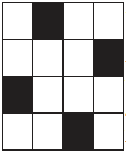### Solution

What is known?

A figure with some part shaded.

What is unknown?

To make the given figure symmetric and diagonal.

Is there more than one way to do that?

Will the figure be symmetric about both the diagonals?

Reasoning:

Take any one diagonal as a line of symmetry and shade a few more squares to make the figure symmetric and diagonal.

Steps: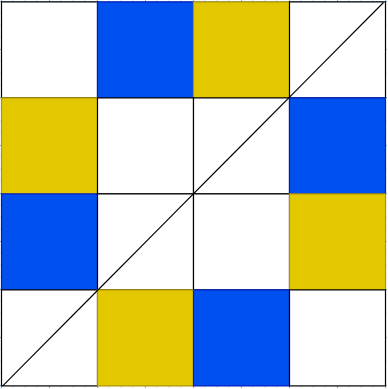Yes, the figure has more than one line of symmetry.

Yes, the figure will be symmetric about both the diagonals.

## Chapter 14 Ex.14.1 Question 6

Copy the diagram and complete each shape to be symmetric about the mirror line(s):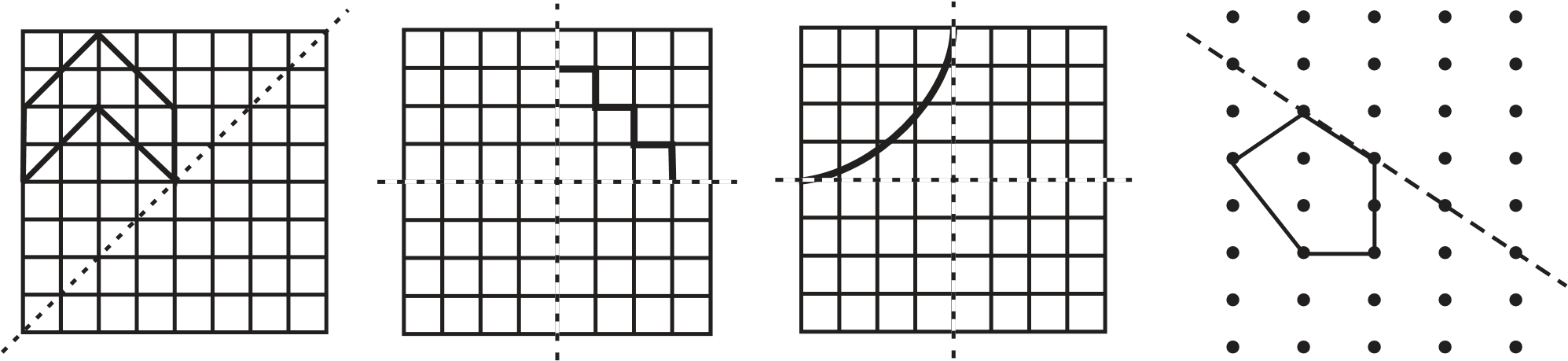### Solution

What is known?

The mirror line (i.e. the line of symmetry) is given as a dotted line.

What is unknown?

Complete each figure performing reflection in the dotted line.

Reasoning:

In this question you have to complete the figure, performing reflection in the dotted (mirror) line. To solve this question, you might perhaps place a mirror along the dotted line and look into the mirror for the image. By doing this you can get the hint which figure is formed and how to complete it.

Steps: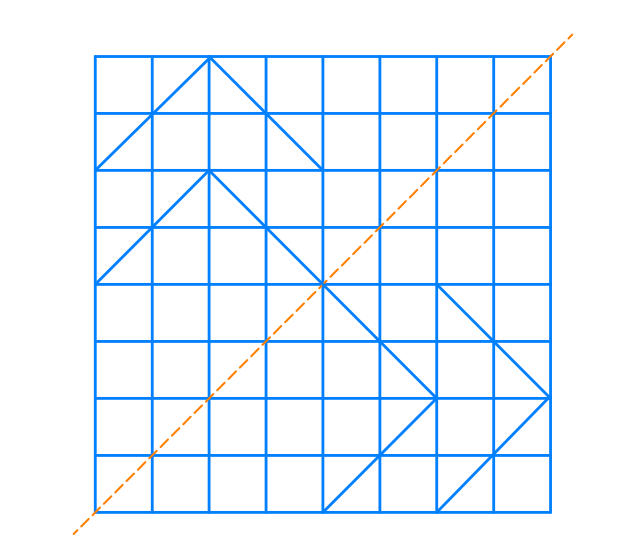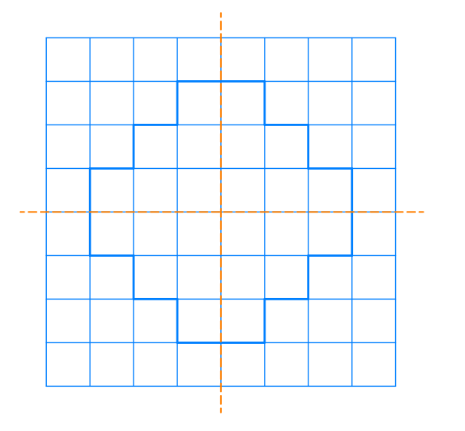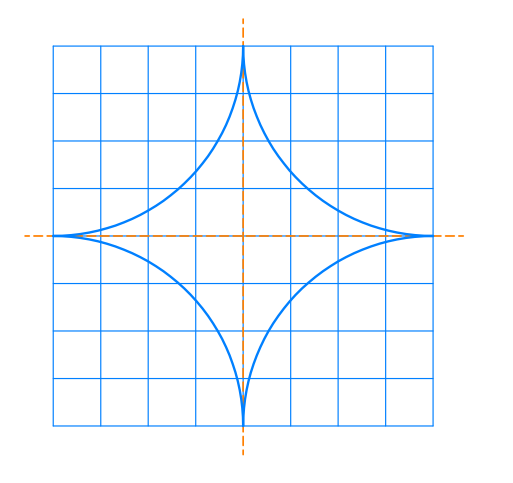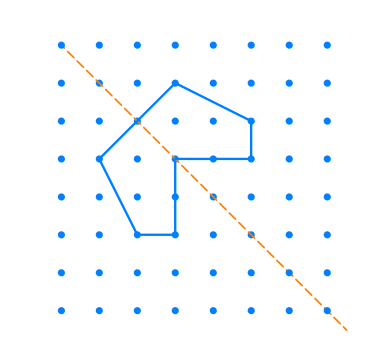## Chapter 14 Ex.14.1 Question 7

State the number of lines of symmetry for the following figures:

(a) An equilateral triangle

(b) An isosceles triangle

(c) A scalene triangle

(d) A square

(e) A rectangle

(f) A rhombus

(g) A parallelogram

(h) A quadrilateral

(i) A regular hexagon

(j) A circle

### Solution

What is known?

Different figures.

What is unknown?

The number of lines of symmetry for the given figures.

Reasoning:

This question is very simple. You have to draw different lines and check which line will divide it into exactly same parts, that line will be called the line of symmetry.

Steps:

(a) It has three lines of symmetry.

(b) It has one line of symmetry.

(c) It has no line of symmetry.

(d) It has four lines of symmetry.

(e) It has two lines of symmetry.

(f) It has two lines of symmetry.

(g) It has no line of symmetry.

(h) It has no line of symmetry.

(i) It has six lines of symmetry.

(j) It has infinitely many lines of symmetry

## Chapter 14 Ex.14.1 Question 8

What letters of the English alphabet have reflectional symmetry (i.e., symmetry related to mirror reflection) about.

(a) vertical mirror

(b) a horizontal mirror

(c) both horizontal and vertical mirrors.

### Solution

What is known:

English alphabets.

What is unknown?

Letters of the English alphabet that have reflectional symmetry

Reasoning:

In this question you have to find out letters of the English alphabet have reflectional symmetry. In reflectional symmetry one half is the reflection of the other half. That is, a figure which does not change upon undergoing a reflection has reflectional symmetry. Remember this concept and find out the letters of English alphabets with reflectional symmetry.

Steps:

(a)  A,H,I,M,O,T,U,V,W,X,Y

(b)  B,C,D,E,H,I,O,X

(c)  O,X,I,H

## Chapter 14 Ex.14.1 Question 9

Give three examples of shapes with no line of symmetry.

### Solution

What is unknown?

Any three shapes with no line of symmetry.

Steps:

A quadrilateral, scalene triangle and a parallelogram.

## Chapter 14 Ex.14.1 Question 10

What other name can you give to the line of symmetry of –

(a) an isosceles triangle?

(b) a circle?

### Solution

What is known?

Name of the shapes

What is unknown?

Another name can you give to the line of symmetry of an isosceles triangle & a circle.

Reasoning:

As it is given in the question, you have to find out the other name for lines of symmetry of an isosceles triangle and a circle. We know that an isosceles triangle has one line of symmetry and this is a line segment joining a vertex to the midpoint of the opposing side, bisecting it. So, we can call it median and a circle has infinitely many lines of symmetry passing through the centre, so we can call it diameter.

Steps:

• Median
• Diameter
Instant doubt clearing with Cuemath Advanced Math Program